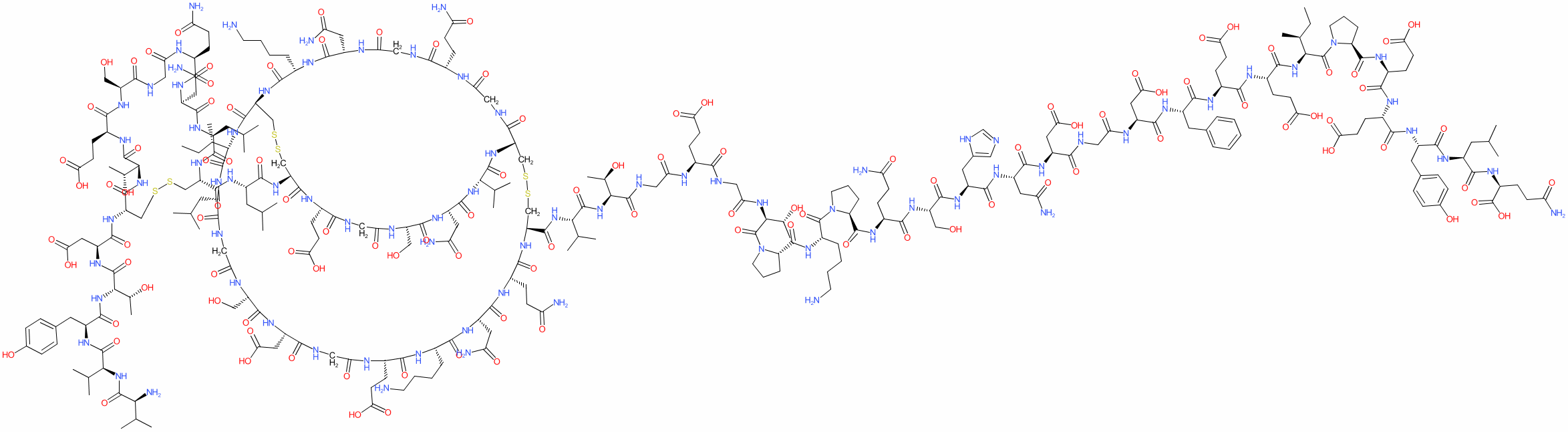#### GtoPdb Ligand ID: 6458

Synonyms: CGP 39393 | CGP-39393 | CGP39393
desirudin is an approved drug (FDA (no history prior to 2003))
Comment: Desirudin is an anticoagulant. The drug is a recombinant form of hirudin (P01050), the salivary anticoagulant found in leeches.

The HELM annotation for desirudin is: PEPTIDE1{V.V.Y.T.D.C.T.E.S.G.Q.N.L.C.L.C.E.G.S.N.V.C.G.Q.G.N.K.C.I.L.G.S.D.G.E.K.N.Q.C.V.T.G.E.G.T.P.K.P.Q.S.H.N.D.G.D.F.View more information in the IUPHAR Pharmacology Education Project: desirudin

2D StructureSMILES / InChI / InChIKeyCanonical SMILES NCCCC[C@@H](C(=O)N1CCC[C@H]1C(=O)N[C@H](C(=O)N[C@H](C(=O)N[C@H](C(=O)N[C@H](C(=O)N[C@H](C(=O)NCC(=O)N[C@H](C(=O)N[C@H](C(=O)N[C@H](C(=O)N[C@H](C(=O)N[C@H](C(=O)N1CCC[C@H]1C(=O)N[C@H](C(=O)N[C@H](C(=O)N[C@H](C(=O)N[C@H](C(=O)N[C@H](C(=O)O)CCC(=O)N)CC(C)C)Cc1ccc(cc1)O)CCC(=O)O)CCC(=O)O)[C@H](CC)C)CCC(=O)O)CCC(=O)O)Cc1ccccc1)CC(=O)O)CC(=O)O)CC(=O)N)Cc1cnc[nH]1)CO)CCC(=O)N)NC(=O)[C@@H]1CCCN1C(=O)[C@H]([C@H](O)C)NC(=O)CNC(=O)[C@@H](NC(=O)CNC(=O)[C@H]([C@H](O)C)NC(=O)[C@H](C(C)C)NC(=O)[C@@H]1CSSC[C@@H]2NC(=O)[C@@H](NC(=O)[C@H](CC(=O)N)NC(=O)[C@H](CO)NC(=O)CNC(=O)[C@H](CCC(=O)O)NC(=O)[C@H](CSSC[C@H](NC(=O)[C@@H](NC(=O)[C@@H](NC(=O)CNC(=O)[C@@H](NC(=O)CNC2=O)CCC(=O)N)CC(=O)N)CCCCN)C(=O)N[C@@H]([C@H](CC)C)C(=O)N[C@H](C(=O)NCC(=O)N[C@H](C(=O)N[C@H](C(=O)NCC(=O)N[C@H](C(=O)N[C@H](C(=O)N[C@H](C(=O)N[C@H](C(=O)N1)CCC(=O)N)CC(=O)N)CCCCN)CCC(=O)O)CC(=O)O)CO)CC(C)C)NC(=O)[C@@H](NC(=O)[C@@H]1CSSC[C@H](NC(=O)[C@@H](NC(=O)[C@H]([C@H](O)C)NC(=O)[C@@H](NC(=O)[C@H](C(C)C)NC(=O)[C@H](C(C)C)N)Cc2ccc(cc2)O)CC(=O)O)C(=O)N[C@@H]([C@H](O)C)C(=O)N[C@@H](CCC(=O)O)C(=O)N[C@H](C(=O)NCC(=O)N[C@H](C(=O)N[C@H](C(=O)N[C@H](C(=O)N1)CC(C)C)CC(=O)N)CCC(=O)N)CO)CC(C)C)C(C)C)CCC(=O)O Isomeric SMILES NCCCC[C@@H](C(=O)N1CCC[C@H]1C(=O)N[C@H](C(=O)N[C@H](C(=O)N[C@H](C(=O)N[C@H](C(=O)N[C@H](C(=O)NCC(=O)N[C@H](C(=O)N[C@H](C(=O)N[C@H](C(=O)N[C@H](C(=O)N[C@H](C(=O)N1CCC[C@H]1C(=O)N[C@H](C(=O)N[C@H](C(=O)N[C@H](C(=O)N[C@H](C(=O)N[C@H](C(=O)O)CCC(=O)N)CC(C)C)Cc1ccc(cc1)O)CCC(=O)O)CCC(=O)O)[C@H](CC)C)CCC(=O)O)CCC(=O)O)Cc1ccccc1)CC(=O)O)CC(=O)O)CC(=O)N)Cc1cnc[nH]1)CO)CCC(=O)N)NC(=O)[C@@H]1CCCN1C(=O)[C@H]([C@H](O)C)NC(=O)CNC(=O)[C@@H](NC(=O)CNC(=O)[C@H]([C@H](O)C)NC(=O)[C@H](C(C)C)NC(=O)[C@@H]1CSSC[C@@H]2NC(=O)[C@@H](NC(=O)[C@H](CC(=O)N)NC(=O)[C@H](CO)NC(=O)CNC(=O)[C@H](CCC(=O)O)NC(=O)[C@H](CSSC[C@H](NC(=O)[C@@H](NC(=O)[C@@H](NC(=O)CNC(=O)[C@@H](NC(=O)CNC2=O)CCC(=O)N)CC(=O)N)CCCCN)C(=O)N[C@@H]([C@H](CC)C)C(=O)N[C@H](C(=O)NCC(=O)N[C@H](C(=O)N[C@H](C(=O)NCC(=O)N[C@H](C(=O)N[C@H](C(=O)N[C@H](C(=O)N[C@H](C(=O)N1)CCC(=O)N)CC(=O)N)CCCCN)CCC(=O)O)CC(=O)O)CO)CC(C)C)NC(=O)[C@@H](NC(=O)[C@@H]1CSSC[C@H](NC(=O)[C@@H](NC(=O)[C@H]([C@H](O)C)NC(=O)[C@@H](NC(=O)[C@H](C(C)C)NC(=O)[C@H](C(C)C)N)Cc2ccc(cc2)O)CC(=O)O)C(=O)N[C@@H]([C@H](O)C)C(=O)N[C@@H](CCC(=O)O)C(=O)N[C@H](C(=O)NCC(=O)N[C@H](C(=O)N[C@H](C(=O)N[C@H](C(=O)N1)CC(C)C)CC(=O)N)CCC(=O)N)CO)CC(C)C)C(C)C)CCC(=O)O InChI Key XYWBJDRHGNULKG-OUMQNGNKSA-N
 ClassificationCompound class Peptide or derivative Approved drug? Yes (FDA (no history prior to 2003))
 International Nonproprietary NamesINN number INN 7193 desirudin
 SynonymsCGP 39393 | CGP-39393 | CGP39393
 Database LinksSpecialist databases Reactome DrugR-ALL-9603260 Reactome ReactionR-HSA-9603302 Other databases CAS Registry No. 120993-53-5 ChEMBL Ligand CHEMBL1201662 GtoPdb PubChem SID 178103073 PubChem CID 16129703 Search Google for chemical match using the InChIKey XYWBJDRHGNULKG-OUMQNGNKSA-N Search Google for chemicals with the same backbone XYWBJDRHGNULKG Search PubMed clinical trials desirudin Search PubMed titles desirudin Search PubMed titles/abstracts desirudin Search UniChem for chemical match using the InChIKey XYWBJDRHGNULKG-OUMQNGNKSA-N Search UniChem for chemicals with the same backbone XYWBJDRHGNULKG